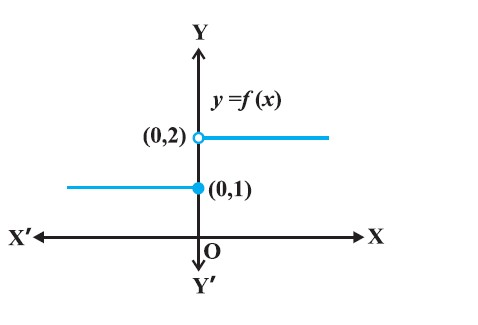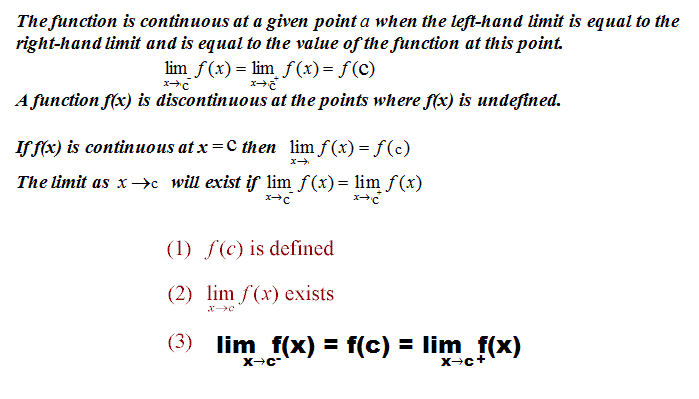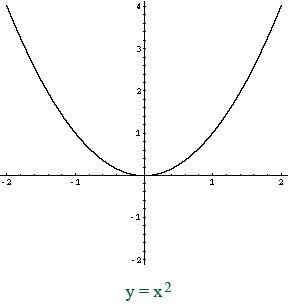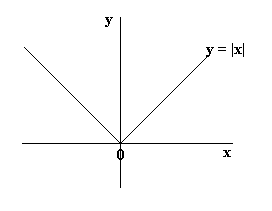Mathematics Introduction, Continuity at a point and Continuity at interval For CBSE-NCERT
Click for Only Video

### Topic covered

star Introduction
star Continuity at a point
starContinuity at interval

### Introduction :Let's Consider the function

f(x)= { tt((1, if , x le 0),(2, if , x > 0 ))

● The value of the function at nearby points on x-axis remain close to each other except at x = 0. At the points near and to the left of 0, i.e., at points like – 0.1, – 0.01, – 0.001, the value of the function is 1.

● At the points near and to the right of 0, i.e., at points like 0.1, 0.01, 0.001, the value of the function is 2.

● Left (respectively right) hand limit of f at 0 is 1 (respectively 2).

● So here the value of the function at x = 0 coincides with the left hand limit.

color{orange}{"Note : we cannot draw this graph in one stroke, i.e., without lifting pen"}
color{orange}{" from the plane of the paper ,we need to lift the pen when we come to "}
color{orange}{0 \ \ " from left. Means function is not continuous at" x = 0.}

### Continuity At a point\color{green} ✍️ If the left hand limit, right hand limit and the value of the function at x = c exist and equal to each other, then f is said to be continuous at x = c.

color{orange}{lim_(x->c^-) f(x) =f(c) = lim_(x->c^+) f(x)}

"● Note :" if the right hand and left hand limits at x = c coincide, its said the common value is the limit of the function at x = c.

● If f is not continuous at c, we say f is discontinuous at c and c is called a point of discontinuity of f.

E.g., Let's examine the function f given by f (x) = x^2 is continuous at x = 0 or not?

● First note that the function is defined at the given point x = 0 and its value is 0.

Then we find the limit of the function at x = 0. ClearlyFor c>0 => lim_(x-> 0^+) f(x) = lim_(x-> 0) x^2=0^2=0
For c<0 => lim_(x-> 0^-) f(x) = lim_(x-> 0) x^2=0^2=0

● Thus lim_(x-> 0) f(x) =0 =f(x)

Hence, f is continuous at x = 0.

Now let's another example

(ii) E.g., the continuity of the function f given by f(x) = | x | at x = 0.

Here, By definition : f(x) ={ tt(( -x, if, x < 0),(x, if , x ge 0))● Clearly the function is defined at 0 and f (0) = 0. Left hand limit of f at 0 is

=> lim_(x-> 0^(-)) f(x) =lim_(x-> 0^(-)) (-x) =0

Similarly, the right hand limit of f at 0 is

=> lim_(x->0^+) f(x) =lim_(x-> 0^+) x=0

Thus, the left hand limit, right hand limit and the value of the function coincide at x = 0. Hence, f is continuous at x = 0.
Q 3155612564Check the continuity of the function f given by f (x) = 2x + 3 at x = 1.
Class 12 Chapter 5 Example 1Solution:

First note that the function is defined at the given point x = 1 and its value is 5.
Then find the limit of the function at x = 1. Clearly

lim_(x->1) f(x) = lim_(x->1) (2x +3) = 2(1) +3 = 5

Thus  lim_(x->1) f(x) = 5 = f (1)

Hence, f is continuous at x = 1.
Q 3125712661Examine whether the function f given by f (x) = x^2 is continuous at x = 0.
Class 12 Chapter 5 Example 2Solution:

First note that the function is defined at the given point x = 0 and its value is 0.
Then find the limit of the function at x = 0. Clearly

 lim_(x->0) f(x) = lim_(x->0) x^2 = 0^2 =0

Thus lim_(x->0) f(x) = 0 = f(0)

Hence, f is continuous at x = 0.
Q 3185712667Discuss the continuity of the function f given by f(x) = | x | at x = 0.
Class 12 Chapter 5 Example 3Solution:

By definition

f(x) = { tt ( ( -x , text (if) x< 0 ), ( x, text (if) x ge 0 ) )

Clearly the function is defined at 0 and f (0) = 0. Left hand limit of f at 0 is

 lim_(x->0^-) f(x) = lim_(x->0^-) (-x) = 0

Similarly, the right hand limit of f at 0 is

lim_(x->0^+) f(x) = lim_(x->0^+) x= 0

Thus, the left hand limit, right hand limit and the value of the function coincide at
x = 0. Hence, f is continuous at x = 0.
Q 3115812760Show that the function f given by

f(x) = { tt ( ( x^3 +3 , text (if) x ≠ 0 ), (1, text(if) x=0) )

is not continuous at x = 0.
Class 12 Chapter 5 Example 4Solution:

The function is defined at x = 0 and its value at x = 0 is 1. When x ≠ 0, the
function is given by a polynomial. Hence,

lim_(x->0) f(x) = lim_(x->0) (x^3 +3) = 0^3 +3 =3

Since the limit of f at x = 0 does not coincide with f (0), the function is not continuous
at x = 0. It may be noted that x = 0 is the only point of discontinuity for this function.
Q 3135812762Check the points where the constant function f (x) = k is continuous.
Class 12 Chapter 5 Example 5Solution:

The function is defined at all real numbers and by definition, its value at any
real number equals k. Let c be any real number. Then

lim_(x->c) f(x) = lim_(x->c) k = k

Since f (c) = k = lim_(x->c) f(x) for any real number c, the function f is continuous at every real number.
Q 3175812766Prove that the identity function on real numbers given by f (x) = x is
continuous at every real number.
Class 12 Chapter 5 Example 6Solution:

The function is clearly defined at every point and f (c) = c for every real
number c. Also,

lim_(x->c) f(x) = lim_(x->c) x = c

Thus  lim_(x->c) f(x) = c = f(c) and hence the function is continuous at every real number.

Having defined continuity of a function at a given point, now we make a natural
extension of this definition to discuss continuity of a function.

### Continuity at an interval :\color{green} ✍️ A real function f is said to be continuous if it is continuous at every point in the domain of f.

\color{green} ✍️ Suppose f is a function defined on a closed interval [a, b], then for f to be continuous, it needs to be continuous at every point in [a, b] including the end points a and b.

=> Continuity of f at a means color{green}{lim_(x-> a^+) f(x) =f(a)}

=> and continuity of f at b means color{green}{lim_(x-> b^(-)) f(x) =f(b)}

\color{green} ✍️ Observe that only lim_(x-> a^(-)) and  lim_(x-> b^(+)) do not make sense. As a consequence of this definition, if f is defined only at one point, it is continuous there, i.e., if the domain of f is a singleton, f is a continuous function.
Q 3115012860Is the function defined by f (x) = | x |, a continuous function?
Class 12 Chapter 5 Example 7Solution:

We may rewrite f as

f(x) = { tt ( ( -x , text (if) x < 0 ), ( x , text (if) x ge 0 ) )

By Example 3, we know that f is continuous at x = 0.
Let c be a real number such that c < 0. Then f (c) = – c. Also

lim_(x->c) f(x) = lim_(x->c) (-x) = -c (Why?)

Since lim_(x->c) f(x) = f(c) , f  is continuous at all negative real numbers.

Now, let c be a real number such that c > 0. Then f (c) = c. Also

lim_(x->c) f(x) = lim_(x->c) x= c (why ? )

Since lim_(x->c ) f (x ) = f(c), f is continuous at all positive real numbers. Hence, f
is continuous at all points.
Q 3145012863Discuss the continuity of the function f given by f (x) = x^3 + x^2 – 1 .
Class 12 Chapter 5 Example 8Solution:

Clearly f is defined at every real number c and its value at c is c^3 + c^2 – 1. We
also know that

lim_(x->c) f(x) = lim_(x->c) (x^3 + x^2 -1) = c^3 + c^2 -1

Thus ` lim_(x->c) f(x) = f(c) , and hence f is continuous at every real number. This means
f is a continuous function.